Want help to update your ClassPad OS? Methods & Spec students - beware of bugs.

Placing these mini-programs into the Library folder on your ClassPad allows access to them not only from Main and the Program application but also from within any eActivity. Watch examples of these mini-programs in the clip below.

### Mini-Program listing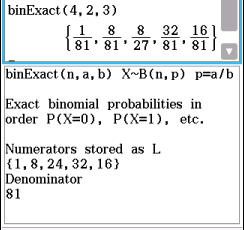binExact is used to display P(X=0), P(X=1) through to P(X=n) in exact form when X~B(n, p). In Main, type in binExact(n,a,b) where n is number of trials and the propability of success p=a/b, so that a is numerator and b is denominator of p in fractional form.

No program for CP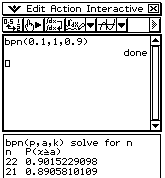bpn is used to find the number of trials (n) needed for P(x ≥ a) ≥ k when X~B(n, p). In Main, type in bpn(p,a,k) where p is probability of success, a is minimum number of successes and k is required propability.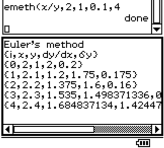emeth applies Euler's method to find an approximate graphical solution to a differential equation (must be explicitly defined), using the incremental formula. In Main, type in emeth(dy/dx,x0,y0,dx,n), where dy/dx is the explicit differential equation, x0 and y0 are the initial conditions, dx is the x-increment and n is the number of iterations required.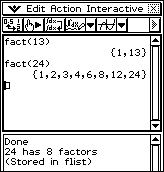fact creates a list of all the factors from 1 to n of any number n. In Main, type in fact(n), where n is the number you want the factors of and they are returned in a list. This list is stored as the variable flist and can be retrieved at any time by typing flist.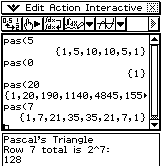pas creates a list of all the coefficients in the nth row of Pascal triangle. In Main, type in pas(n), where n is the row you want and the coefficients are returned in a list. This list is stored as the variable plist and can be retrieved at any time by typing plist.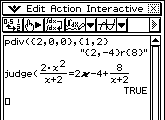pdiv is used to divide one polynomial by another (of equal or lower order). Enter coefficients of numerator and denominator as lists (curly brackets) and the quotient and remainder are returned.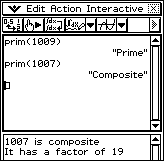prim checks to see whether any number n is prime. In Main, type in prim(n), where n is the number you want to check.

Note: OS 3.04 introduced the new IsPrime function, which is similar to this function but returns TRUE or FALSE.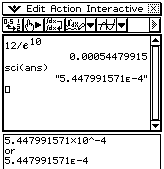sci takes any number and returns it in scientific notation (standard form). In Main type in sci(number).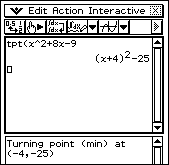tpt takes a quadratic expression in any form and returns it in turning point (completed square) form. In Main type in tpt(expression), where expression is the quadratic you want to find the turning point of.

Also visit the Programs and Functions pages.

### News

Free and paid tutorials at ClassPad Help and the Tuition Centre sites.

Enrol in an online course with Charlie at The Tuition Centre

Buy practice ATAR exams from WA Exam Papers

Check out how WA schools stand in the latest SCSA rankings

© 2023 Charlie Watson. PO Box 366, SUBIACO, WA 6904.